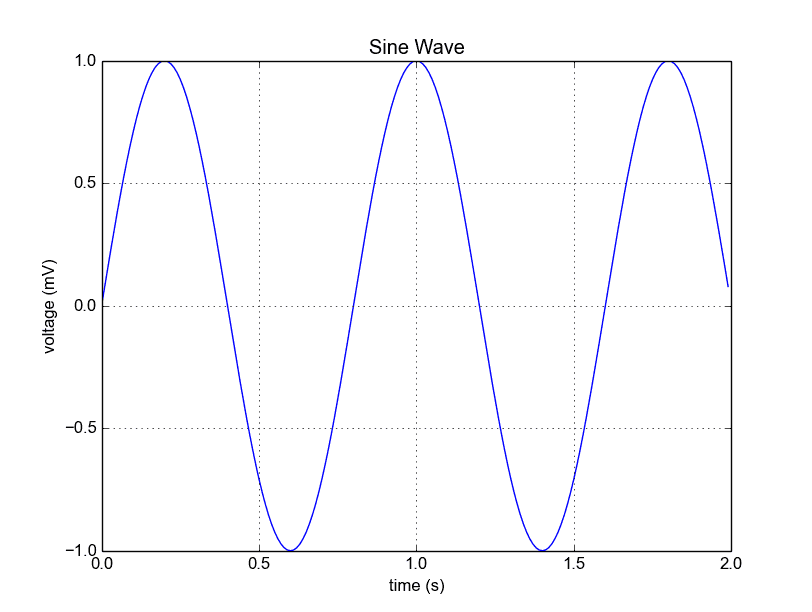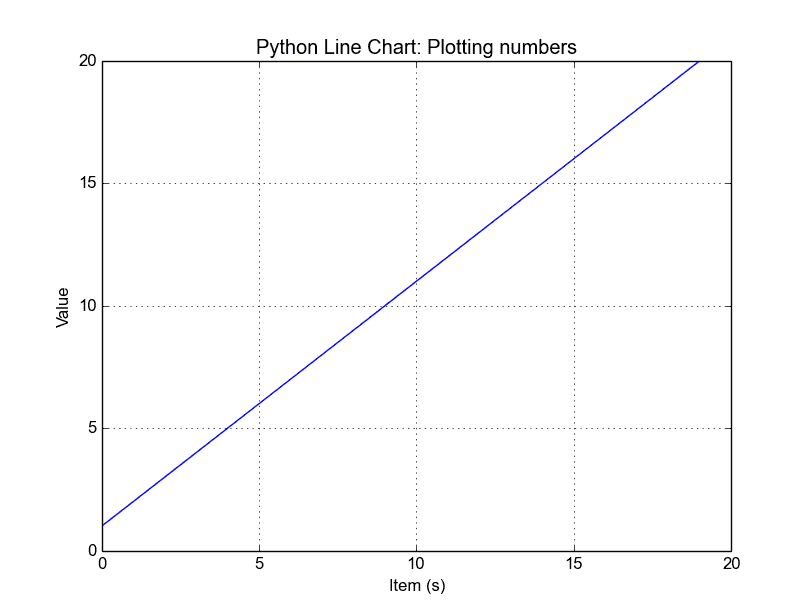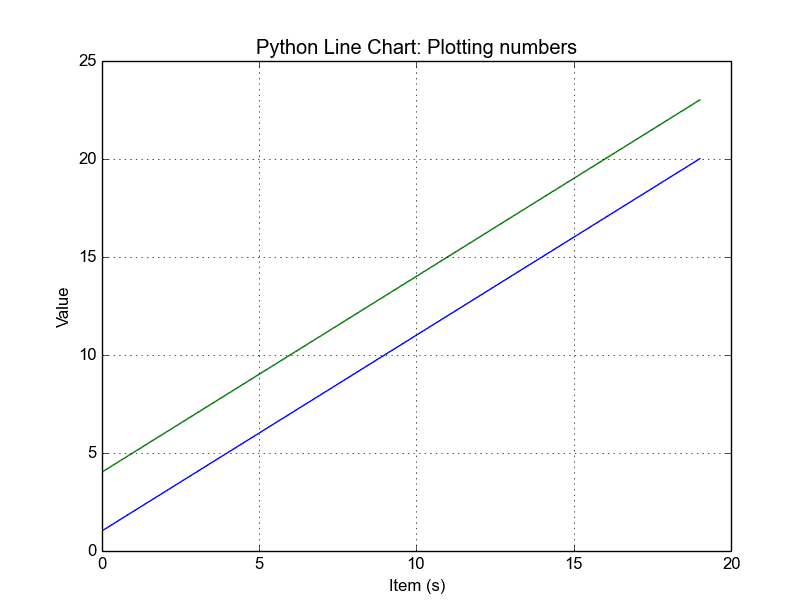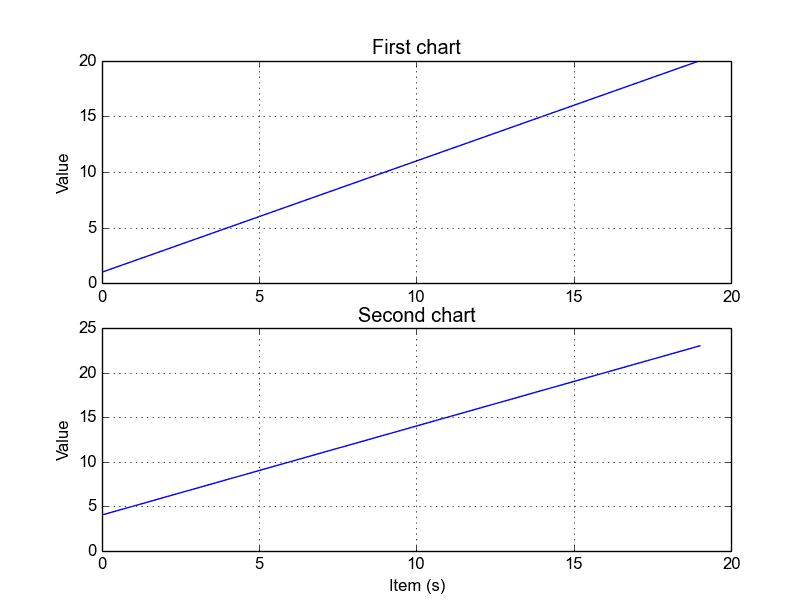# Tag: linechart

## Matplotlib Line chart

A line chart can be created using the Matplotlib plot() function. While we can just plot a line, we are not limited to that. We can explicitly define the grid, the x and y axis scale and labels, title and display options.

Related course:

Line chart example
The example below will create a line chart.

Output:Python Matplotlib Line Chart

The lines:

simply define the data to be plotted.

plots the chart.  The other statements are very straightforward: statements xlabel() sets the x-axis text, ylabel() sets the y-axis text, title() sets the chart title and grid(True) simply turns on the grid.

If you want to save the plot to the disk, call the statement:

Plot a custom Line Chart

If you want to plot using an array (list), you can execute this script:

The statement:

defines start from 0, plot 20 items (length of our array) with steps of 1.

Output:Python Line Chart from List

Multiple plots

If you want to plot multiple lines in one chart, simply call the plot() function multiple times. An example:

Output:python line chart multiple

In case you want to plot them in different views in the same window you can use this:

Output:Python subplots

The plt.subplot() statement is key here. The subplot() command specifies numrows, numcols and fignum.

Styling the plot
If you want thick lines or set the color, use: